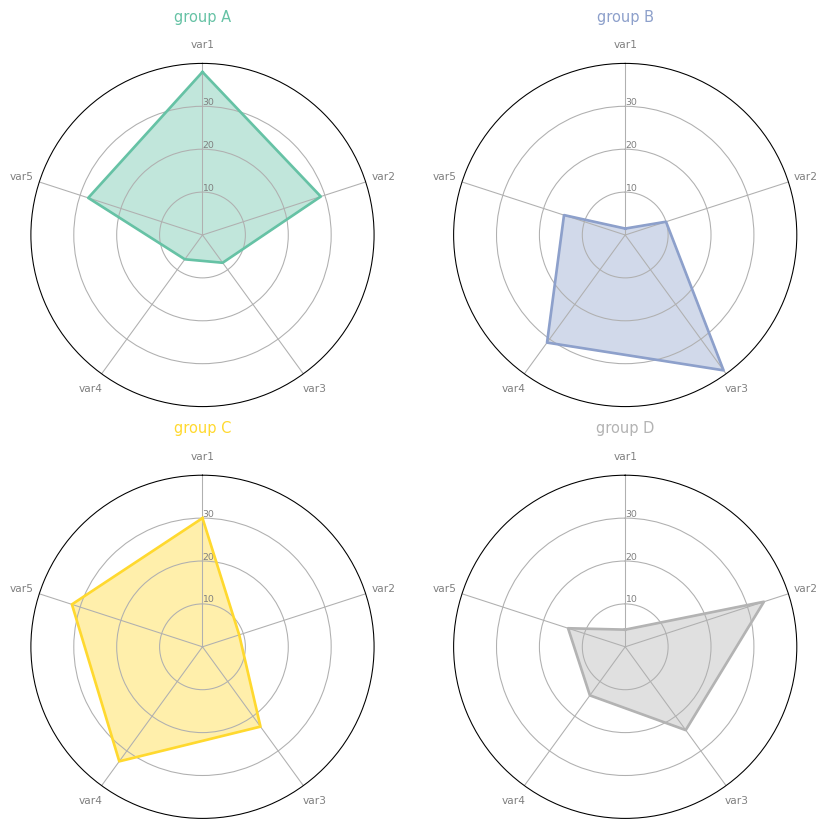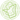# Use faceting for Radar chart

The posts #390 and #391 showed how to make a radar chart with one or two individuals. This post describes using faceting when displaying more than two individuals on a radar chart using matplotlib.

It is not advised to display too many entities on the same spider plot since it would result in an unreadable plot. Instead, you may prefer faceting. When you display each individual in a separate plot, the figure stays clear and the reader can compare the shape of each plot easily.

Here is the code allowing to make this figure. Do not hesitate to read this page to understand how faceting works with matplotlib.

``````# Libraries
import matplotlib.pyplot as plt
import pandas as pd
from math import pi

# Set data
df = pd.DataFrame({
'group': ['A','B','C','D'],
'var1': [38, 1.5, 30, 4],
'var2': [29, 10, 9, 34],
'var3': [8, 39, 23, 24],
'var4': [7, 31, 33, 14],
'var5': [28, 15, 32, 14]
})

# ------- PART 1: Define a function that do a plot for one line of the dataset!

def make_spider( row, title, color):

# number of variable
categories=list(df)[1:]
N = len(categories)

# What will be the angle of each axis in the plot? (we divide the plot / number of variable)
angles = [n / float(N) * 2 * pi for n in range(N)]
angles += angles[:1]

# Initialise the spider plot
ax = plt.subplot(2,2,row+1, polar=True, )

# If you want the first axis to be on top:
ax.set_theta_offset(pi / 2)
ax.set_theta_direction(-1)

# Draw one axe per variable + add labels labels yet
plt.xticks(angles[:-1], categories, color='grey', size=8)

# Draw ylabels
ax.set_rlabel_position(0)
plt.yticks([10,20,30], ["10","20","30"], color="grey", size=7)
plt.ylim(0,40)

# Ind1
values=df.loc[row].drop('group').values.flatten().tolist()
values += values[:1]
ax.plot(angles, values, color=color, linewidth=2, linestyle='solid')
ax.fill(angles, values, color=color, alpha=0.4)

plt.title(title, size=11, color=color, y=1.1)

# ------- PART 2: Apply the function to all individuals
# initialize the figure
my_dpi=96
plt.figure(figsize=(1000/my_dpi, 1000/my_dpi), dpi=my_dpi)

# Create a color palette:
my_palette = plt.cm.get_cmap("Set2", len(df.index))

# Loop to plot
for row in range(0, len(df.index)):
make_spider( row=row, title='group '+df['group'][row], color=my_palette(row))``````## Contact & Edit

👋 This document is a work by Yan Holtz. Any feedback is highly encouraged. You can fill an issue on Github, drop me a message onTwitter, or send an email pasting `yan.holtz.data` with `gmail.com`.